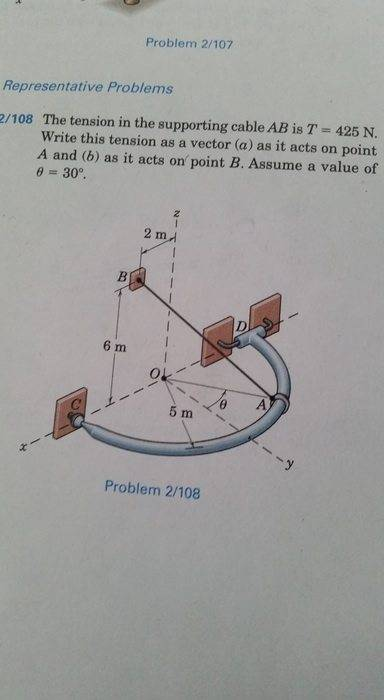# Find tension as a vector on point A and point B1. Homework Statement

The tension in the supporting cable AB is T = 425 N. Write this tension as a vector (a) as it acts on point A and (b) as it acts on point B. Assume θ = 30°. [***I've attached a picture of the exact problem]

The answer in my text book says TA = <221, -212, 294>
TB = <-221, 212, -294>

## Homework Equations

T [/B]= (T)(n)

n
= (AB) / (||AB||)

## The Attempt at a Solution

I started by finding the AB which I used A = (0, 5cos(30), 5sin(30)) and B = (2, 0, 6), which gave me AB = <2, -4.33, 3.5>.

Next I used n = (AB) / (||AB||) to find n = <.338, -.732, .5916>

Then I found T = (T)(n) = <143.65, -311.1, 251.43>

I'm not sure what I'm doing wrong here. The only thing I can think of is if I'm using incorrect points for point A

Astronuc
Staff Emeritus
It looks like θ is in the x-y (azimuthal) plane, so it should not apply directly to the z-coordinate as one did for the coordinates of A, necessarily.

One needs to think in terms of two angles, poloidal and azimuthal. Note point B is offset from the polar axis.

It looks like θ is in the x-y (azimuthal) plane, so it should not apply directly to the z-coordinate as one did for the coordinates of A, necessarily.

One needs to think in terms of two angles, poloidal and azimuthal. Note point B is offset from the polar axis.

Thank you! I'm pretty sure fixed my problem. I absolutely hate when the text book doesn't make it obvious which plane the angle is in.

So that changed my A to A = (-2.5, 4.33, 0), which changed my tension to the correct answer.